## Wednesday, 11 December 2013

### Area Under Curve (AUC) - pROC package

One of the useful methods of comparing performance of statistical models is Area Under Curve (AUC). The area under curve here refers to area under ROC curve. ROC curve stands for Receiver Operating Characteristics. This was first used during World War II to display performance of a radar system. In general, ROC curve is drawn against True Positive Rate (sensitivity) and False Positive Rate (inverse of specificity i.e. false positive rate = 1 - specificity).

There are several ways of calculating AUC, but one of the easiest way is to use pROC package. The below example shows how to calculate AUC of a rpart model.

library(rpart) library(pROC) Dat <- kyphosis a <- rep(0, nrow(Dat)) a[which(Dat\$Kyphosis == "present")] <- 1 Dat\$Present <- a a <- sample(c(1:nrow(Dat)), size = nrow(Dat) * 0.7, replace = FALSE) Train <- Dat[a, ] Test <- Dat[-a, ] RP1 <- rpart(Present ~ Age + Number + Start, Train, control = rpart.control(minsplit = 15, cp = 1e-04)) Pred1 <- predict(RP1, Test[, c("Age", "Number", "Start")]) Test\$Prediction1 <- Pred1 ROC1 <- roc(Test\$Present, Test\$Prediction1) plot(ROC1, col = "blue")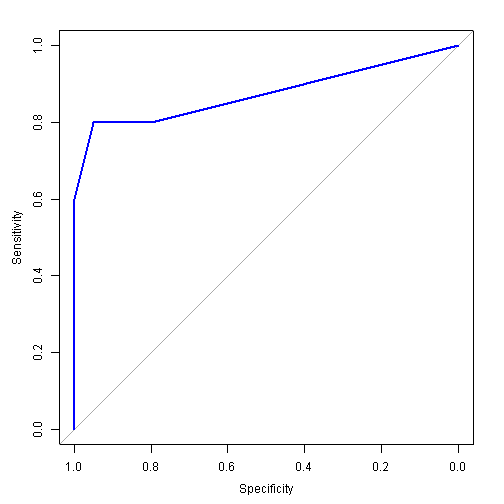AUC1 <- auc(ROC1) AUC1

## Area under the curve: 0.875

In the above example, as the curve moves away from the grey diagonal line towards top left corner of the graph, sensitivity and specificity increase, and at the same time, the area under the graph increases. Hence, the models with higher performance will show the ROC curve closer to the top left corner, increasing the area under curve.

To compare with the above result, a larger decision tree is developed from the same dataset that would have an overfitting effect.

RP2 <- rpart(Present ~ Age + Number + Start, Train, control = rpart.control(minsplit = 3, cp = 1e-05)) opar <- par(mfrow = c(1, 2)) plot(RP1, main = "RP1") plot(RP2, main = "RP2")
par(opar)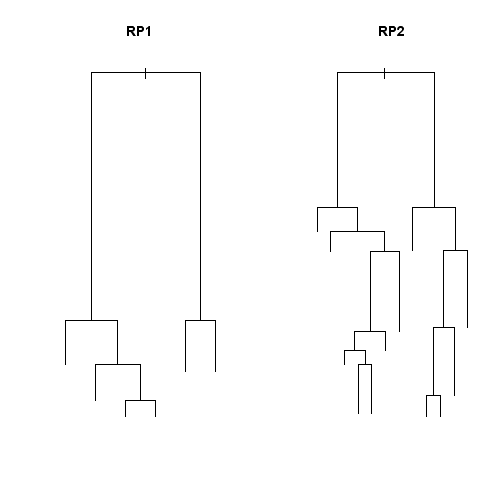The below is the ROC and AUC for the larger tree.

Pred2 <- predict(RP2, Test[, c("Age", "Number", "Start")]) Test\$Prediction2 <- Pred2 ROC2 <- roc(Test\$Present, Test\$Prediction2) plot(ROC1, col = "blue")
par(new = TRUE) plot(ROC2, col = "green", xaxt = "n", yaxt = "n")
legend("right", legend = c("RP1", "RP2"), col = c("blue", "green"), lty = 1)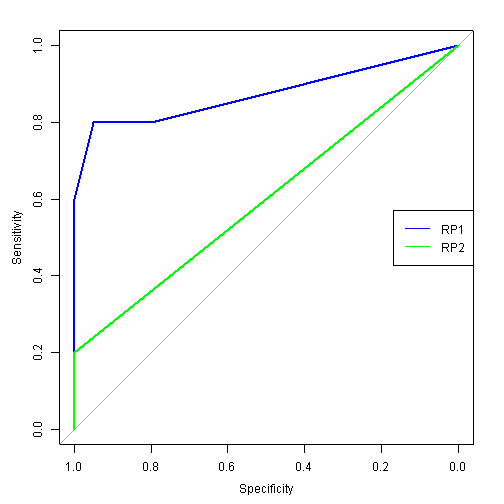As expected the larger tree (RP2) overfit the data and produced poorer performance shown by ROC curve appearing closer to the grey diagonal line.

The below AUC calculation supports the above observation. This example shows clear difference between the performance of the two models without the AUC calculation but in other instances, the graphs could be closer together or intersect with each other, making it hard to judge. The calculation of AUC becomes useful in such circumstances.

AUC1

## Area under the curve: 0.875

AUC2 <- auc(ROC2) AUC2

## Area under the curve: 0.6

Perhaps, AUC becomes most useful when conducting cross-validation. There will be too many ROC curves to properly compare the model performances, and comparing error rates at a certain threshold point does not reflect the overall model performance. Hence, AUC becomes a useful indicator as shown below.
SUMM <- data.frame()
n <- 0 repeat { n <- n + 1 b <- sample(c(1:nrow(Test)), size = nrow(Test) * 0.8, replace = FALSE) TDat <- Test[b, ] Pred1 <- predict(RP1, TDat[, c("Age", "Number", "Start")]) Pred2 <- predict(RP2, TDat[, c("Age", "Number", "Start")]) TDat\$Score1 <- Pred1 TDat\$Score2 <- Pred2 ROC1 <- roc(TDat\$Present, TDat\$Score1) AUC1 <- auc(ROC1) SUMMX <- data.frame(validation = n, model = "RP1", AUC = AUC1) SUMM <- rbind(SUMM, SUMMX) ROC2 <- roc(TDat\$Present, TDat\$Score2) AUC2 <- auc(ROC2) SUMMX <- data.frame(validation = n, model = "RP2", AUC = AUC2) SUMM <- rbind(SUMM, SUMMX) if (n == 10) { break } } boxplot(AUC ~ model, SUMM, ylim = c(0, 1))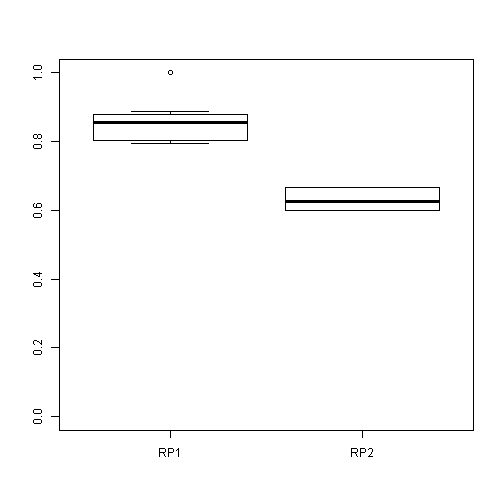In this example, boxplot is used to plot AUCs of each model obtained during cross validation. This shows the accuracy as well as the robustness of the model, and the difference between the performances of two models is now more apparent.

#### 5 comments:

1.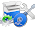Programming is very interesting and creative thing if you do it with love. Your blog code helps a lot to beginners to learn programming from basic to advance level. I really love this blog because I learn a lot from here and this process is still continuing.
Love from Pro Programmer

1.Thank you for your kind words!

2.Great post dear. It definitely has increased my knowledge on R Programming. Please keep sharing similar write ups of yours. You can check this too for R Programming tutorial as i have recorded this recently on R Programming. and i'm sure it will be helpful to you.https://www.youtube.com/watch?v=rgFVq_Q6VF0

3.I appreciate your work on Data Science. It's such a wonderful read on Data Science course. Keep sharing stuffs like this. I am also educating people on similar Data Science training so if you are interested to know more you can watch this Data Science tutorial:-https://www.youtube.com/watch?v=gXb9ZKwx29U&t=237s

4.Very Impressive ROC Curve Data Science tutorial. The content seems to be pretty exhaustive and excellent and will definitely help in learning ROC Curve Data Science course. I'm also a learner taken up ROC Curve Data Science training and I think your content has cleared some concepts of mine. While browsing for ROC Curve Data Science tutorials on YouTube i found this fantastic video on ROC Curve Data Science. Do check it out if you are interested to know more.:-https://www.youtube.com/watch?v=G_pvQYUm8Ik